• 首先要明白一个前提，这里介绍的是没有使用神经网络的qlearning，而不是Deep QLearning。而且算法不使用梯度下降，而是迭代更新。 一、算法详解 1.1 几个概念 1.1.1 什么是critic？ critic：批评家，评论家。 在这里...
一、算法详解
文章最后附有博主自己实现的深度qlearning玩space invader游戏本文介绍的是基于神经网络的qlearning算法。我们知道传统的qlearning算法只能处理状态和动作有限的情况，对于无穷多，则是无法有效处理的。现实生活中，环境的状态肯定是无穷多的，而神经网络正好可以处理这样的情况。这里深度qlearning算法使用一个神经网络来表示一个q表，无论环境的状态有没有出现过，我们都可以将状态输入到神经网络，去评估价值函数。
1.1 几个概念
1.1.1 什么是critic？
critic：批评家，评论家。
在这里算法要更新就是一个critic，而不是一个actor（agent），使用critic来间接指导actor做决策，critic的作用就是评估一个actor有多好，水平高不高。
1.1.2 状态价值函数$V ^{\pi }(s)$和状态动作价值函数$Q^{\pi }(s,a)$,以及他们之间的关系
$\pi$表示一个actor，s是一个状态，a是指actor看到状态s时做出的动作

$V ^{\pi }(s)$指使用actor  $\pi$时，当看到状态s时，计算累计奖励的期望值
举个例子吧，不然有点难以理解，这里计算的依然是累计奖励的期望值。我个人理解，如果要写出具体 $V ^{\pi }(s)$公式的话，应该是这样
$V^{\pi}(s)=\sum_{\tau }R(\tau ,s)p(\tau)\tag{1}$
公式解释：$R(\tau ,s)$表示在一个eposide内，只计算s之后的所有累计奖励的和。比如$\tau =\{s_{1},a_{1},r_{1},s_{2},a_{2},r_{2},...,s_{T},a_{T},r_{T},End\}$,我们计算$R(\tau ,s_{2})$,由上面的定义知道，$R(\tau ,s_{2})=r_{2}+...+r_{T}$,这里没有计算$r_{1}$,只计算看到$s_{2}$之后的所有奖励。$p(\tau)$表示eposide $\tau$出现的概率。
举一个sutton强化学习书上的例子。
例子1：
假设我们采样得到8个eposides,分别如下：
1、$s_{a},r=0,s_{b},r=0,End$
2、$s_{b},r=1,End$
3、$s_{b},r=1,End$
4、$s_{b},r=1,End$
5、$s_{b},r=1,End$
6、$s_{b},r=1,End$
7、$s_{b},r=1,End$
8、$s_{b},r=0,End$
这里给出了8个采样得到的eposide，并且忽略所采取的动作，这里是采样得到8个eposide来逼近$V^{\pi}(s)$,选择采样来逼近$V^{\pi}(s)$，是因为actor所处的环境和actor本身都具有随机性，由上面的公式（1）可以看到，如果所有的eposides有无穷多个，那么计算机根本无法实现计算$V^{\pi}(s)$
根据上面的8个eposides，可以计算得到：
$V^{\pi}(s_{b})=\frac{1+1+1+1+1+1}{8}=\frac{3}{4}$
只有在第二到第七个eposide时，遇到状态$s_{b}$才有奖励，第一个eposide中，当遇到状态$s_{b}$时，所有累积的奖励值是0。第8个eposide也是如此。就算第一个eposide变成$s_{a},r=1,s_{b},r=0,End$，$V^{\pi}(s)$依然不变还是$\frac{3}{4}$.

$Q^{\pi }(s,a)$指actor看到状态$s$之后确定选择动作$a$之后的奖励累积期望
为什么说确定选择动作$a$呢？看到状态$s$,其实有很多个动作可以选择，但是在这里就是确定选择动作$a$,而不是别的动作。还有一个因素就是选择动作的时候具有随机性，比如采用$\varepsilon -greedy$方法，会有一定的几率随机选择动作。

两者之间的关系
假设有$...,s_{t},a_{t},r_{t},s_{t+1},...$,那么就有$Q^{\pi }(s_{t},a_{t})=E(r_{t}+V ^{\pi }(s_{t+1}))$
这里还是要求期望的。不过在实际操作的时候需要采样或者直接把变成$Q^{\pi }(s_{t},a_{t})=r_{t}+V ^{\pi }(s_{t+1})$。这种情况就是只采样一个用来逼近期望。这样网络收敛的效果可能不是太好，毕竟用一条样本来逼近还是效果不大好的。

1.1.3 如何计算$V ^{\pi }(s)$？

Monte-Carlo(MC)方法
如下图，我们使用一个神经网络来计算$V ^{\pi }(s)$，并且根据actor玩游戏的实际情况，来优化这个神经网络，并且利用这个神经网络（q表，我们前面说到了使用神经网络来表示这个q表以处理状态极其复杂多变的情况，所以神经网络==q表）来指导actor进行决策。例子：假设有状态 $s_{a}和 s_{b}$,计算$V ^{\pi }(s_{a})和V ^{\pi }(s_{b})$的步骤如下：
对于$V ^{\pi }(s_{a})$:
(1) actor（agent）玩游戏或者和环境互动.
(2) 状态 $s_{a}$ 出现在某个回合（episode）中.
(3) 仅记录从状态 $s_{a}$ 出现到这个回合结束后的累积的奖励，我们将其记为$G_{a}$.
(4) 将状态$s_{a}$输入到神经网络然后输出 $V ^{\pi }(s_{a})$,这个神经网络输出的是个标量.
(5) 利用 回归(regression) 的方法来优化神经网络，使得神经网络的输出的$V ^{\pi }(s_{a})$来逼近$G_{a}$.
(6) 对于任何一个状态都可以重复以上过程，直到神经网络收敛。
对于$V ^{\pi }(s_{b})$的计算也是如此。

Temporal-Difference-Approach(时间差分方法)
时间差分方法，顾名思义，肯定是需要两个连续时间步上的状态才能达到训练神经网络的目的。
假设actor玩游戏或者与环境互动的一个episode中的连续两步是$...,s_{t},a_{t},r_{t},s_{t+1},...$
首先，$V^{\pi }(s_{t})=r_{t}+V^{\pi }(s_{t+1})\tag{2}$
${\pi}$表示agent或者actor。
时间差分方法每连续的两个时间步都会训练一次神经网络，因此收敛的速度也会相对来说较快。步骤如下
(1) 假设在时间步 $t$ 观测到状态 $s_{t}$
(2) actor根据现在的状态 $s_{t}$ 做出动作 $a_{t}$ ,得到奖励 $r_{t}$
(3) actor观测到下一个时间步 $t+1$ 的状态 $s_{t+1}$
(4) 将状态 $s_{t}$, $s_{t+1}$ 输入进神经网络得到 $V^{\pi }(s_{t})$ 和 $V^{\pi }(s_{t+1})$
(5) 利用公式2，我们将$V^{\pi }(s_{t})$ 和 $V^{\pi }(s_{t+1})$的差值逼近时间步的奖励 $r_{t}$. 还是利用回归(Regression)的方法来训练神经网络。图片来自李宏毅老师的强化学习课程，侵删！！！

两种方法之间的关系
蒙特卡洛方法有更大的方差，方差大说明效果不好，相比来说时间差分方法的训练速度更加高效，收敛的更快，时间差分方法只需要使用两个时间步的信息就可以训练网络，而蒙特卡洛方法却需要等待一个完整的episode完成之后才可以进行训练。不知道大家发现没有，突然觉得这个方法也是有点类似监督学习方法，这个深度qlearning算法里面的“监督信息是环境反馈过来的奖励”，我们需要使用奖励来指引actor的学习。
对于同一个采样出来的样本，使用两种方法计算出来的同一个状态 $s_{a}$ 的价值函数是不一样的
比如下面的例子：
假设我们采样得到8个eposides,分别如下：
1、$s_{a},r=0,s_{b},r=0,End$
2、$s_{b},r=1,End$
3、$s_{b},r=1,End$
4、$s_{b},r=1,End$
5、$s_{b},r=1,End$
6、$s_{b},r=1,End$
7、$s_{b},r=1,End$
8、$s_{b},r=0,End$
我们先使用蒙特卡洛方法计算 $V^{\pi}(s_{a})$:
观察采样出来的数据，我们可以看到状态 $s_{a}$ 只是出现在 第一条样本里面，而且直到游戏结束，得到的两个奖励值都是0，所以 $G_{a}=0$,因为我们需要让 $V^{\pi}(s_{a})$ 逼近 $G_{a}$,所以理想情况下有$V^{\pi}(s_{a})=0$.
接着使用时间差分方法来计算$V^{\pi}(s_{a})$：
从第一条采样样本可以看到状态 $s_{a}$ 和 $s_{b}$ 是两个相邻的状态，因此由公式2可以知道计算 $V^{\pi}(s_{a})$，需要使用 $V^{\pi}(s_{b})$的值。
根据采样的8条样本，我们使用这八条样本的 \$V^{\pi}(s_{b})_{i},i=1,2,…,8的期望值来近似逼近 $V^{\pi}(s_{b})$
因此有$V^{\pi}(s_{b})=\frac{1+1+1+1+1+1}{8}=\frac{3}{4}$
由在第一条样本中在状态 $s_{a}$ 时actor得到的奖励是0，所以$V^{\pi }(s_{a})=0+V^{\pi }(s_{b})=\frac{3}{4}$.
可以看到，不同的计算方法得到的价值函数的值是不一样的。

二、算法运行流程
步骤一： actor(agent) ${\pi}$和环境（Environment）互动
步骤二： 使用蒙特卡洛方法或者时间差分方法来计算状态价值函数$V^{\pi}(s)$或者状态动作价值函数$Q^{\pi}(s,a)$
步骤三： 使用回归（Regression）方法训练神经网络
步骤四： 价值函数指导actor做出更好的策略，循环以上步骤，直到收敛。
基于TD方法的QLearning具体如下图：算法流程里面的butter要改为buffer。
三、几个小技巧
3.1 技巧1 target网络和predict网络
其实在传统的qlearning里面以及涉及到了“q_target和q_predict”的概念了，这里的深度qlearning在训练的时候同样也是由target网络和predict网络，只不过是两个网络需要共享参数，其中target是固定的，只有在predict网络以target的输出为目标更新若干次之后采后将predict网络的参数重新赋给target网络。
有需要可以看看传统的qlearning算法。传送门：传统qlearning算法讲解
我们以李宏毅老师的课程讲解如何训练的这里使用的是时间差分方法来训练的
右边的 Target 网络的参数在一定时间内是固定的，Target网络输出的值是左边网络需要回归的目标，然后更新这个来更新左边的predict网络，更新若干次之后，然后将predict网络的参数重新赋给Target网络，一直训练，直到收敛。
3.2、技巧2之 $\varepsilon -greedy$选择动作
使用这个技巧有利于actor学会探索，也可以确保当训练的次数足够多时，所有的动作都可以被的更新到。随机探索的可能性会随着训练的进行逐渐变小的
我们之前在强化学习（RL）QLearning算法详解介绍过了这个技巧，不再重复。
3.3 技巧3 Boltzmann选择动作
利用状态动作价值函数的大小来选择动作，值越大，这个对于的动作被选择的概率就越大，对于的动作被选择的概率公式如下：
$P(a|s)=\frac{exp(Q(s,a))}{\sum_{a}exp(Q(s,a))}\tag{3}$
3.4 技巧4 RePlay Buffer
设计一个Buffer将actor玩过的experience存储起来，可以重复使用这个数据更新网络。
假如使用时间差分方法，我们可以在Buffer存储器里面存储只需要两个时间步就可以，比如其中一条数据可以是：$s_{t},a_{t},r_{t},s_{t+1}$,当储存很多时，也可以进行batch学习。
四、小例子
基于卷积神经网络的小例子
4.1 readme

安装gym
安装atari-py
在第二步很容易出现ale_c.dll不存在的问题。
以下是解决方法
分三步：
第一步：先卸载atari-py。pip uninstall atari-py
第二步：再重新安装这个。pip install --no-index -f https://github.com/Kojoley/atari-py/releases atari_py
第三步：pip install gym

# -*- coding: utf-8 -*-

import gym
import torch.nn as nn
import torch as t
from torch.nn import functional as F
import random

dicount_factor = 0.9
eplison = 0.1
lr = 0.001
epochs = 50
nums_p2t = 100 #每隔100词将Q_Net_predict的参数赋给Q_Net_target,然后继续固定target网络
env = gym.make("SpaceInvaders-v0") # 构造一个太空入侵者的环境

# 下面这个神经网络是用来预测
class Q_Net_predict(nn.Module):
def __init__(self, nums_action):
super(Q_Net_predict,self).__init__()
#下面开始定义卷积和全连接层，计划使用两个全连接层和两个卷积层
self.conv1 = nn.Conv2d(3, 16, 5, 2)
self.conv2 = nn.Conv2d(16, 16, 5, 2)

self.linear1 = nn.Linear(1728,256)
self.linear2 = nn.Linear(256,nums_action)

def forward(self, x):

#先进行类型的转换
state = t.from_numpy(x[:,:,::-1].copy())
state = state.permute((2,0,1)).unsqueeze(dim=0).float()

#开始使用卷积，最大池化和线性层
out = self.conv1(state)
out = F.relu(out)
out = F.max_pool2d(out,(2,2))

out = self.conv2(out)
out = F.relu(out)
out = F.max_pool2d(out,(2,2))
s = out.size()

out = out.view(1,s*s*s)

out = F.relu(self.linear1(out))
out = self.linear2(out)
return out

# 下面这个神经网络是用来作为Q_Net_predict拟合的目标函数
class Q_Net_target(nn.Module):
def __init__(self, nums_action):
super(Q_Net_target,self).__init__()
#下面开始定义卷积和全连接层，计划使用两个全连接层和两个卷积层
self.conv1 = nn.Conv2d(3, 16, 5, 2)
self.conv2 = nn.Conv2d(16, 16, 5, 2)

self.linear1 = nn.Linear(1728,256)
self.linear2 = nn.Linear(256,nums_action)

def forward(self, x):

#先进行类型的转换
state = t.from_numpy(x[:,:,::-1].copy())
state = state.permute((2,0,1)).unsqueeze(dim=0).float()

#开始使用卷积，最大池化和线性层
out = self.conv1(state)
out = F.relu(out)
out = F.max_pool2d(out,(2,2))

out = self.conv2(out)
out = F.relu(out)
out = F.max_pool2d(out,(2,2))
s = out.size()

out = out.view(1,s*s*s)

out = F.relu(self.linear1(out))
out = self.linear2(out)
return out

def choose_action(logits):
## 使用eplison-greedy选择agent需要执行的动作
v = random.uniform(0, 1)
q_value, index = t.topk(logits, 1, dim = 1)

#下面开始eplison-greedy 算法
if v > eplison:

#这里是求最大的状态价值函数对应的动作
q_value_t = logits[0,index]
action = index.item()

else:
#下面是随机产生动作
action = random.randint(0, 5)
q_value_t = logits[0, action]
return action, q_value_t

def q_learning():

all_count = 0
#下面开始
#先定义两个状态价值函数网络
q_target = Q_Net_target(6)
q_predict = Q_Net_predict(6)

#定义一个优化器
opt_Adam = t.optim.Adam(q_predict.parameters(),lr = lr)
#将target网络的参数冻结
for p in q_target.parameters():
p.requires_grad = False

for _ in range(epochs):
done = False
#初始化一个状态
observation = env.reset() #每个episode的初始状态
while not done:
env.render()

#下面开始网络的参数复制
if all_count % nums_p2t == 0:
target_paras = q_target.state_dict()
predict_paras = q_predict.state_dict()

target_paras.update(predict_paras)
q_target.load_state_dict(target_paras)
#下面使用q_predict网络的输出选择动作
predict_logits = q_predict(observation)
action, q_value_t = choose_action(logits= predict_logits)

#下面根据动作得到奖励以及下一个时间步的状态observation
observation, reward, done, info = env.step(action)

#现在有了observation,需要使用使用target网络计算observation的状态价值函数
target_qvalue = q_target(observation)
q_value_t_ = max(target_qvalue).item()

#我们需要使reward+q_value_t_ 和 q_value_t接近
loss = (reward + q_value_t_ - q_value_t)**2
loss.backward()
opt_Adam.step()
all_count +=1
env.close()

if __name__ == '__main__':
q_learning()


五、参考文献
1、李宏毅老师的强化学习算法
2、莫烦python的强化学习系列
3、ale_c.dll确实解决方法
4、openai官网


展开全文强化学习 机器学习 神经网络 python
• 这篇文章将要介绍传统的qlearning算法，使用的是迭代的方法更新q表，更新q表的方法类似于向前推进，而不是使用梯度下降方法，因为这里介绍的不是Deep QLearning方法。 一、算法介绍以及推导 注：这里更新的不是agent...
注意将代码和下面公式推导结合起来。还要注意一下q_target和q_predict之间的关系。其实算法的更新是需要使用q_predict来逼近q_target，当两者相等时，算法将停止更新，当传统的qlearning转化为deep Qlearning,也是这样操作的，只是深度qlearning使用一个神经网络来表示q表。
这篇文章将要介绍传统的qlearning算法，使用的是迭代的方法更新q表，更新q表的方法类似于向前推进，而不是使用梯度下降方法，因为这里介绍的不是Deep QLearning方法。
一、算法介绍以及推导
注：这里更新的不是agent，而是一个q表，q表里面记录的是agent在某个状态采取某个的动作的好坏，q表可以起到间接决定agent采取什么决策。q表就类似一个critic，一个评论家，来指导agent。
1.1、$Q(s,a)$是什么？
$Q(s,a)$是状态动作价值函数，是在状态$s$时采取动作$a$之后，可以获得的奖励的期望值。$Q(s,a)$越大表示在agent在看到状态$s$是采取动作$a$比较好。
1.2、q表

q表
a1
a2
a3

s1
q(s1,a1)
q(s1,a2)
q(s1,a3)

s2
q(s2,a1)
q(s2,a2)
q(s2,a3)

s3
q(s3,a1)
q(s3,a2)
q(s3,a3)

s4
q(s4,a1)
q(s4,a2)
q(s4,a3)

q表里面记录的都是状态动作价值函数，前面说到q表可以间接决定agent采取什么样的决策，就是因为q表记录了所有的状态和动作的组合情况，比如agent看到状态$s_{2}$时，就会在状态$s_{2}$所在的行选取最大的q值所对应的动作。
1.3、如何根据q表进行决策？
假设下表是我们已经更新完成了的q表

q表
a1
a2
a3

s1
- 1
1
3

s2
2
0
1

s3
1
5
7

s4
5
6
3

Q表指导agent决策的过程：t=1时，agent观测到环境的状态s2，于是查找状态s2所在的行，发现Q(s2,a1)>Q(s2,a3)>Q(s2,a2),因此选择动a1,此时环境发生变化，agent观测到环境的状态s4，接着查找状态s4所在的行，agent发现q(s4,a2)>q(s4,a1)>q(s4,a3)，于是agent采取决策选择动作a2，一直进行下去，直到结束。
1.4、$\varepsilon -greedy$选择动作
有上面的决策过程我们可以看到，在给定一个状态s时，会选择q值最大的动作，这样会导致一个问题：因为是随机初始化的，由于选择最大值，可能会使得一些动作无法被选择到，也就是无法更新q值，这样q值就一直是随机初始化的那个值。
$\varepsilon -greedy$选择动作的流程如下：agent观测到状态s时，采取动作时会以$1-\varepsilon$的概率在Q表里面选择q值最大所对应的动作，以$\varepsilon$的概率随机选择动作。
不再使用完全贪婪的算法，而是有一定的动作选择的完全随机性，这样就可以保证在迭代次数足够多的情况下Q表中的所有动作都会被更新到。
1.5、如何更新Q表？
算法开始的时候，我们需要随机初始化q表，那么如何更新q表就是一件非常关键的事情。参数解释：
更新公式为$Q(s,a) ^{new} = Q(s,a) ^{old}+\alpha [r+\gamma max_{{a}'}Q({s}',{a}')-Q(s,a)^{old}]\tag{1}$

$\alpha$指学习率，其实也是一个权值，我们将公式1进行改写得到如下的公式$Q(s,a) ^{new} = (1-\alpha )Q(s,a) ^{old}+\alpha [r+\gamma max_{{a}'}Q({s}',{a}')]\tag{2}$
我们从公式2可以看到，$Q(s,a) ^{new}$是两部分的凸组合。

$\gamma$ 是衰减值

首先我们随机初始化一个Q表，然后任意初始化一个状态$s$，也可以理解为，agent观测到的环境的状态，根据Q表使用$\varepsilon -greedy$算法选择状态$s$对应的动作$a$,因为agent做出了一个动作，会从环境中获得一个奖励 $r$，环境发生变化，agent又观测到一个新的状态 ${s}'$，根据Q表在状态 ${s}'$ 所在的行，查询Q表，求得最大值，然后更新按照公式更新Q表。
Question 1：什么时候才会迭代收敛？
答：由公式（1）可以看到当  $r+\gamma max_{{a}'}Q({s}',{a}')==Q(s,a)^{old}$时，迭代格式收敛，也就是Q表的更新完成。
1.6、TD方法（时间差分更新法）
假设$...,s_{t},a_{t},r_{t},s_{t+1},...$
我们要更新Q值使 $r_{t}和Q(s_{t},a_{t})^{old}-\gamma max_{{a}'}Q(s_{t+1},{a}')$越接近越好，这里用了两个相邻时刻的状态价值函数
二、两个个小疑问

等eposide结束之后，进行反向更新
从公式 $Q(s,a) ^{new} = Q(s,a) ^{old}+\alpha [r+\gamma max_{{a}'}Q({s}',{a}')-Q(s,a)^{old}]$ 可以看到，$Q(s,a)$的更新会使用到状态
${s}'$的Q值，从而基于TD方法进行更新Q表。但是每次更新我们都利用了下一个时刻的Q值，比如$Q({s}',{a}')$，假设agent完了一个episode的游戏，得到$\tau =\{s_{1},a_{1},r_{1},s_{2},a_{2},r_{2},...,s_{T},a_{T},r_{T},END\}$,假如我们先从后面的状态开始更新，这样前面的状态更新的时候就可以使用已经更新了的后面的状态，也就是反向更新
不知道这样是否可行，这样有个问题就是：原始的方法每一时间步都可以更新，就是在episode进行的时候更新，换成上面我说的这样的更新方法，还要将状态，动作，价值先存储起来，要等到episode结束才能更新。

agent观测一个状态，能不能选择做出多个动作回应这个状态？
例子：太空入侵者游戏在这个游戏中，agent可以做出动作有三种：开火（fire）,向右移动（right）,向左移动（left）.
假设上图为agent观测到的一个状态，但是这是agent同时选择了开火和向右移动的动作。感觉这样也是合理的，只要做出的动作不相互矛盾就可以。比如动作向右移动和向左移动就不能同时发生。

三、代码
import numpy as np
import pandas as pd
import time

np.random.seed(2)  # reproducible

N_STATES = 6   # the length of the 1 dimensional world
ACTIONS = ['left', 'right']     # available actions
EPSILON = 0.9   # greedy police
ALPHA = 0.1     # learning rate
GAMMA = 0.9    # discount factor
MAX_EPISODES = 13   # maximum episodes
FRESH_TIME = 0.3 # fresh time for one move

'''
所构造的q表的大小是：行数是财产的探索者总共所处的位置数，列数就是动作的数量
'''
def build_q_table(n_states, actions):
table = pd.DataFrame(
np.zeros((n_states, len(actions))),     # q_table initial values
columns=actions,    # actions's name
)
# print(table)    # show table
return table

def choose_action(state, q_table):
# This is how to choose an action
state_actions = q_table.iloc[state, :] #将现在agent观测到的状态所对应的q值取出来
if (np.random.uniform() > EPSILON) or ((state_actions == 0).all()):  #当生撑随机数大于EPSILON或者状态state所对应的q值全部为0时，就随机选择状态state所对应的动作
action_name = np.random.choice(ACTIONS)
else:   # act greedy
action_name = state_actions.idxmax()    # 选择状态state所对应的使q值最大的动作
return action_name

def get_env_feedback(S, A):
# 选择动作之后，还要根据现在的状态和动作获得下一个状态，并且返回奖励，这个奖励是环境给出的，用来评价当前动作的好坏。
#这里设置的是，只有在获得宝藏是才给奖励，没有获得奖励时，无论是向左移动还是向右移动，给出的即时奖励都是0.
if A == 'right':    # move right
if S == N_STATES - 2:   # terminate
S_ = 'terminal'
R = 1
else:
S_ = S + 1
R = 0
else:   # move left
R = 0
if S == 0:
S_ = S  # reach the wall
else:
S_ = S - 1
return S_, R

def update_env(S, episode, step_counter):
# 更新环境的函数，比如向右移动之后，o表示的agent就距离宝藏进了一步，将agent随处的位置实时打印出来
env_list = ['-']*(N_STATES-1) + ['T']   # '---------T' our environment
if S == 'terminal':
interaction = 'Episode %s: total_steps = %s' % (episode+1, step_counter)
print('\r{}'.format(interaction), end='')
time.sleep(2)
print('\r                                ', end='')
else:
env_list[S] = 'o'
interaction = ''.join(env_list)
print('\r{}'.format(interaction), end='')
time.sleep(FRESH_TIME)

def rl():
# 开始更新q表
q_table = build_q_table(N_STATES, ACTIONS)#随机初始化一下q表
for episode in range(MAX_EPISODES):
step_counter = 0#记录走了多少步
S = 0#每个episode开始时都将agent初始化在最开始的地方
is_terminated = False
update_env(S, episode, step_counter)#打印的就是o-----T
while not is_terminated:#判断episode是否结束

A = choose_action(S, q_table)#agent根据当前的状态选择动作
S_, R = get_env_feedback(S, A)  # 上一步已经获得了s对应的动作a，接着我们要获得下一个时间步的状态
q_predict = q_table.loc[S, A]
if S_ != 'terminal':#要判断一下，下一个时间步的是不是已经取得宝藏了，如果不是，可以按照公式进行更新
q_target = R + GAMMA * q_table.iloc[S_, :].max()   # next state is not terminal
else:#如果已经得到了宝藏，得到的下一个状态不在q表中，q_target的计算也不同。
q_target = R     # next state is terminal
is_terminated = True    # terminate this episode

q_table.loc[S, A] += ALPHA * (q_target - q_predict)  # update
S = S_  # move to next state

update_env(S, episode, step_counter+1)
step_counter += 1
return q_table

if __name__ == "__main__":
q_table = rl()
print('\r\nQ-table:\n')
print(q_table)

可以参看下面的链接
莫烦python


展开全文人工智能 强化学习
• ## 【强化学习】Q-Learning算法详解

万次阅读 多人点赞 2018-06-19 21:18:18
QLearning是强化学习算法中值迭代的算法，Q即为Q（s,a）就是在某一时刻的 s 状态下(s∈S)，采取 a (a∈A)动作能够获得收益的期望，环境会根据agent的动作反馈相应的回报reward r，所以算法的主要思想就是将State与...
【强化学习】Q-Learning详解

https://morvanzhou.github.io/tutorials/machine-learning/reinforcement-learning/2-1-general-rl/ 莫凡大神的有趣的强化学习视频通俗易懂

发现了很多RL资料搬砖过来，刚入门的可以用得上

David Silver 博士的 UCL 公开课：http://www0.cs.ucl.ac.uk/staff/d.silver/web/Teaching.html
DeepMind 和 UCL 的DL、RL课程：https://www.youtube.com/playlist?list=PLqYmG7hTraZDNJre23vqCGIVpfZ_K2RZs
Sergey Levine 的DRL课程：http://rail.eecs.berkeley.edu/deeprlcourse/
OpenAI 的 Spinning Up in Deep RL：https://blog.openai.com/spinning-up-in-deep-rl/
关于深度强化学习良心paper：https://arxiv.org/abs/1810.06339

1、算法思想
QLearning是强化学习算法中value-based的算法，Q即为Q（s,a）就是在某一时刻的 s 状态下(s∈S)，采取 动作a (a∈A)动作能够获得收益的期望，环境会根据agent的动作反馈相应的回报reward r，所以算法的主要思想就是将State与Action构建成一张Q-table来存储Q值，然后根据Q值来选取能够获得最大的收益的动作。

Q-Table
a1
a2

s1
q(s1,a1)
q(s1,a2)

s2
q(s2,a1)
q(s2,a2)

s3
q(s3,a1)
q(s3,a2)

2、公式推导
举个例子如图有一个GridWorld的游戏从起点出发到达终点为胜利掉进陷阱为失败。智能体（Agent）、环境状态（environment）、奖励（reward）、动作（action）可以将问题抽象成一个马尔科夫决策过程，我们在每个格子都算是一个状态 $s_t$ , π(a|s)在s状态下采取动作a策略 。 P(s’|s,a)也可以写成$P_{ss'}^a$为在s状态下选择a动作转换到下一个状态s’的概率。R(s’|s,a)表示在s状态下采取a动作转移到s’的奖励reward，我们的目的很明确就是找到一条能够到达终点获得最大奖赏的策略。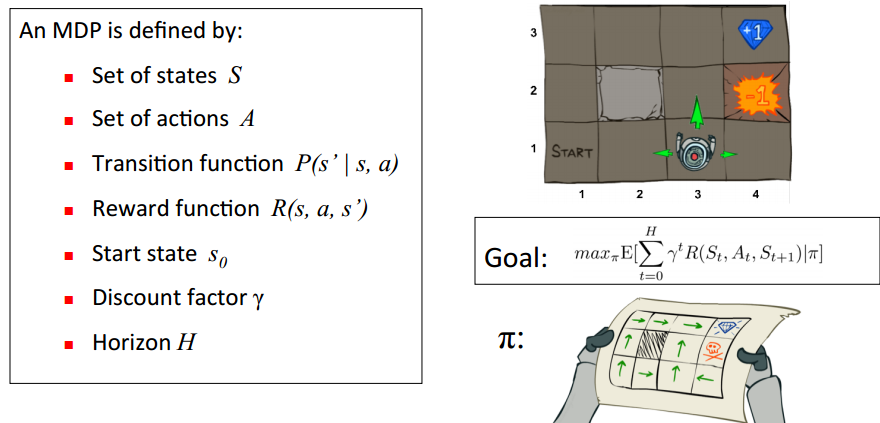所以目标就是求出累计奖励最大的策略的期望：
Goal：$\max_πE[\sum_{t=0}^{H}γ^tR(S_t,A_t,S_{t+1}) | π]$
Qlearning的主要优势就是使用了时间差分法TD（融合了蒙特卡洛和动态规划）能够进行离线学习, 使用bellman方程可以对马尔科夫过程求解最优策略
贝尔曼方程
通过bellman方程求解马尔科夫决策过程的最佳决策序列，状态值函数$V_\pi(s)$可以评价当前状态的好坏，每个状态的值不仅由当前状态决定还要由后面的状态决定，所以状态的累计奖励求期望就可得出当前s的状态值函数V(s)。bellman方程如下
$V_π(s) = E(U_t|S_t = s)$
$V_π(s) = E_π[R_{t+1}+γ[R_{t+2} + γ[.......]]|S_t = s]$
$V_π(s) = E_π[R_{t+1}+γV(s')|S_t = s]$
最优累计期望可用$V^*(s)$表示，可知最优值函数就是$V^*(s)=max_πV_\pi(s)$
$V^*(s)=\max_πE[\sum_{t=0}^{H}γ^tR(S_t,A_t,S_{t+1}) | π,s_0=s]$
Q(s,a)状态动作值函数
$q_π(s,a) = E_π[r_{t+1}+γr_{t+2}+γ^2r_{t+3}+....|A_t=a,S_t=s]$
$q_π(s,a) = E_π[G_t|A_t=a,S_t=s]$
其中$G_t$是t时刻开始的总折扣奖励，从这里我们能看出来 γ衰变值对Q函数的影响，γ越接近于1代表它越有远见会着重考虑后续状态的的价值，当γ接近0的时候就会变得近视只考虑当前的利益的影响。所以从0到1，算法就会越来越会考虑后续回报的影响。
$q_π(s,a) = E_π[R_{t+1}+γq_π(S_{t+1},A_{t+1})|A_t=a,S_t=s]$
最优价值动作函数$Q^*(s,a)=max_\pi Q^*(s,a)$,打开期望如下
$Q^*(s,a)=\sum_{s'} P(s'|s,a)(R(s,a,s')+γ\max_{a'}Q^*(s',a'))$
Bellman方程实际上就是价值动作函数的转换关系
$V_π(s) = \sum_{a∈A}π(a|s)q_π(s,a)$
$q_π(s,a) = R_s^a + γ\sum_{s'∈S}P_{ss'}^aV_π(s')$
$V_π(s)=\sum_{a'∈A}π(a|s)[R_s^a+γ\sum_{s'}P_{ss'}^aV_π(s')]$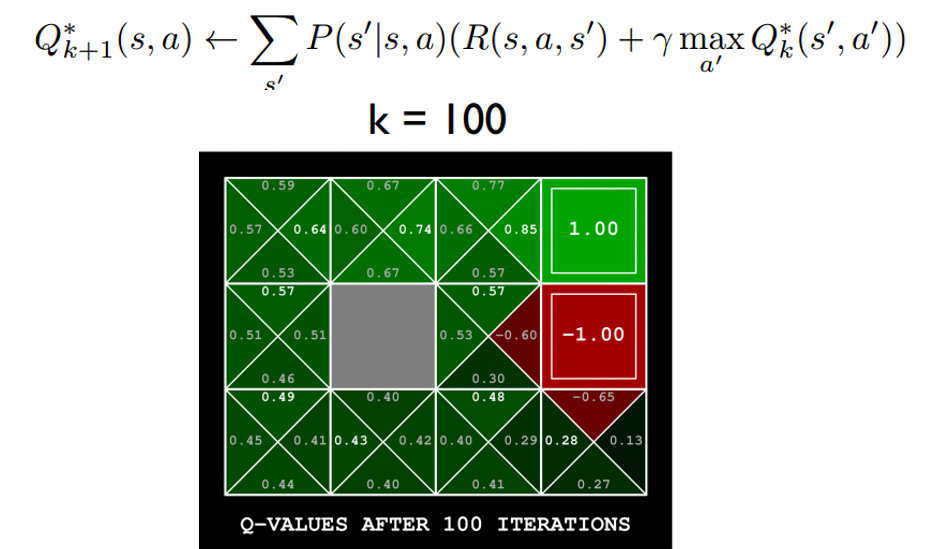根据下图更直观的了解V(s)与Q(s,a)的关系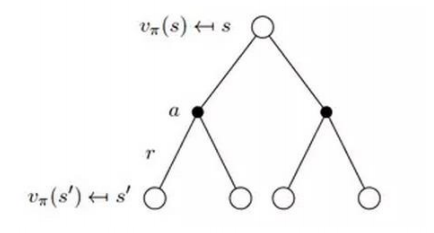时间差分法 https://blog.csdn.net/qq_30615903/article/details/80821061
时间差分方法结合了蒙特卡罗的采样方法和动态规划方法的bootstrapping(利用后继状态的值函数估计当前值函数)使得他可以适用于model-free的算法并且是单步更新，速度更快。值函数计算方式如下
$V(s)←V(s)+\alpha (R_{t+1}+\gamma V(s')-V(s))$
其中$R_{t+1}+\gamma V(s')$被称为TD目标，$\delta_t=R_{t+1}+\gamma V(s')-V(s)$  称为TD偏差。
3、更新公式
根据以上推导可以对Q值进行计算，所以有了Q值我们就可以进行学习，也就是Q-table的更新过程，其中α为学习率γ为奖励性衰变系数，采用时间差分法的方法进行更新。
$Q(s,a) ← Q(s,a) + α[r + γmax_{a'}Q(s',a')-Q(s,a)]$
上式就是Q-learning更新的公式，根据下一个状态s’中选取最大的$Q(s',a')$值乘以衰变γ加上真实回报值最为Q现实，而根据过往Q表里面的Q(s,a)作为Q估计。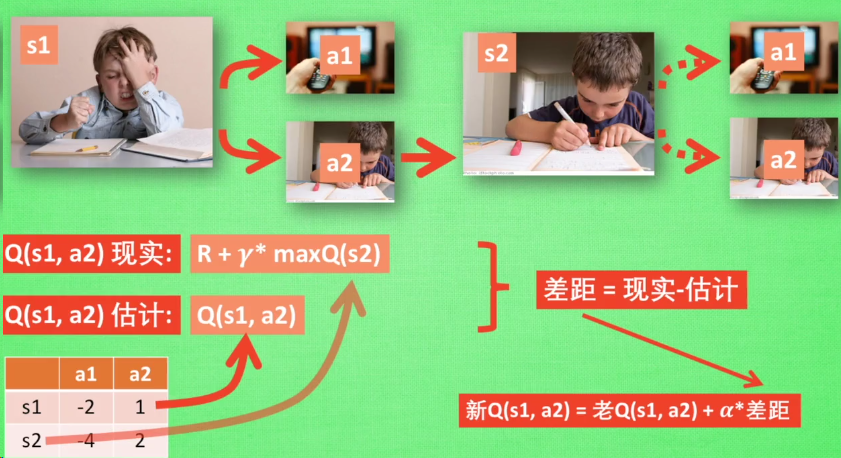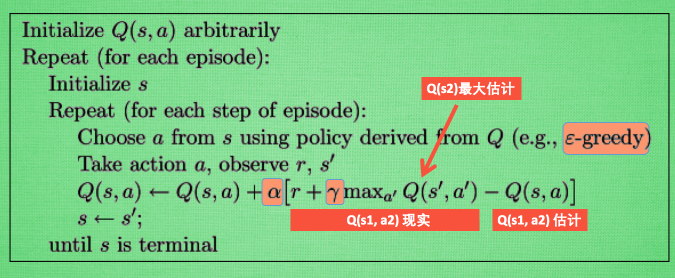4、实现代码
代码来自网上各路大神的源码，非原创，据反映没图片跑不通，所以建了个github，https://github.com/xshura/reinforcement_learning
Q-Learning agent
# -*- coding: utf-8 -*-
import random
from environment import Env
from collections import defaultdict

class QLearningAgent:
def __init__(self, actions):
# actions = [0, 1, 2, 3]
self.actions = actions
self.learning_rate = 0.01
self.discount_factor = 0.9
self.epsilon = 0.1
self.q_table = defaultdict(lambda: [0.0, 0.0, 0.0, 0.0])

# 采样 <s, a, r, s'>
def learn(self, state, action, reward, next_state):
current_q = self.q_table[state][action]
# 贝尔曼方程更新
new_q = reward + self.discount_factor * max(self.q_table[next_state])
self.q_table[state][action] += self.learning_rate * (new_q - current_q)

# 从Q-table中选取动作
def get_action(self, state):
if np.random.rand() < self.epsilon:
# 贪婪策略随机探索动作
action = np.random.choice(self.actions)
else:
# 从q表中选择
state_action = self.q_table[state]
action = self.arg_max(state_action)
return action

@staticmethod
def arg_max(state_action):
max_index_list = []
max_value = state_action
for index, value in enumerate(state_action):
if value > max_value:
max_index_list.clear()
max_value = value
max_index_list.append(index)
elif value == max_value:
max_index_list.append(index)
return random.choice(max_index_list)

if __name__ == "__main__":
env = Env()
agent = QLearningAgent(actions=list(range(env.n_actions)))
for episode in range(1000):
state = env.reset()
while True:
env.render()
# agent产生动作
action = agent.get_action(str(state))
next_state, reward, done = env.step(action)
# 更新Q表
agent.learn(str(state), action, reward, str(next_state))
state = next_state
env.print_value_all(agent.q_table)
# 当到达终点就终止游戏开始新一轮训练
if done:
break

环境部分
import time
import numpy as np
import tkinter as tk
from PIL import ImageTk, Image

np.random.seed(1)
PhotoImage = ImageTk.PhotoImage
UNIT = 100
HEIGHT = 5
WIDTH = 5

class Env(tk.Tk):
def __init__(self):
super(Env, self).__init__()
self.action_space = ['u', 'd', 'l', 'r']
self.n_actions = len(self.action_space)
self.title('Q Learning')
self.geometry('{0}x{1}'.format(HEIGHT * UNIT, HEIGHT * UNIT))
self.shapes = self.load_images()
self.canvas = self._build_canvas()
self.texts = []

def _build_canvas(self):
canvas = tk.Canvas(self, bg='white',
height=HEIGHT * UNIT,
width=WIDTH * UNIT)
# create grids
for c in range(0, WIDTH * UNIT, UNIT):  # 0~400 by 80
x0, y0, x1, y1 = c, 0, c, HEIGHT * UNIT
canvas.create_line(x0, y0, x1, y1)
for r in range(0, HEIGHT * UNIT, UNIT):  # 0~400 by 80
x0, y0, x1, y1 = 0, r, HEIGHT * UNIT, r
canvas.create_line(x0, y0, x1, y1)

# add img to canvas
self.rectangle = canvas.create_image(50, 50, image=self.shapes)
self.triangle1 = canvas.create_image(250, 150, image=self.shapes)
self.triangle2 = canvas.create_image(150, 250, image=self.shapes)
self.circle = canvas.create_image(250, 250, image=self.shapes)

# pack all
canvas.pack()

return canvas

def load_images(self):
rectangle = PhotoImage(
Image.open("../img/rectangle.png").resize((65, 65)))
triangle = PhotoImage(
Image.open("../img/triangle.png").resize((65, 65)))
circle = PhotoImage(
Image.open("../img/circle.png").resize((65, 65)))

return rectangle, triangle, circle

def text_value(self, row, col, contents, action, font='Helvetica', size=10,
style='normal', anchor="nw"):
if action == 0:
origin_x, origin_y = 7, 42
elif action == 1:
origin_x, origin_y = 85, 42
elif action == 2:
origin_x, origin_y = 42, 5
else:
origin_x, origin_y = 42, 77

x, y = origin_y + (UNIT * col), origin_x + (UNIT * row)
font = (font, str(size), style)
text = self.canvas.create_text(x, y, fill="black", text=contents,
font=font, anchor=anchor)
return self.texts.append(text)

def print_value_all(self, q_table):
for i in self.texts:
self.canvas.delete(i)
self.texts.clear()
for i in range(HEIGHT):
for j in range(WIDTH):
for action in range(0, 4):
state = [i, j]
if str(state) in q_table.keys():
temp = q_table[str(state)][action]
self.text_value(j, i, round(temp, 2), action)

def coords_to_state(self, coords):
x = int((coords - 50) / 100)
y = int((coords - 50) / 100)
return [x, y]

def state_to_coords(self, state):
x = int(state * 100 + 50)
y = int(state * 100 + 50)
return [x, y]

def reset(self):
self.update()
time.sleep(0.5)
x, y = self.canvas.coords(self.rectangle)
self.canvas.move(self.rectangle, UNIT / 2 - x, UNIT / 2 - y)
self.render()
# return observation
return self.coords_to_state(self.canvas.coords(self.rectangle))

def step(self, action):
state = self.canvas.coords(self.rectangle)
base_action = np.array([0, 0])
self.render()

if action == 0:  # up
if state > UNIT:
base_action -= UNIT
elif action == 1:  # down
if state < (HEIGHT - 1) * UNIT:
base_action += UNIT
elif action == 2:  # left
if state > UNIT:
base_action -= UNIT
elif action == 3:  # right
if state < (WIDTH - 1) * UNIT:
base_action += UNIT

# 移动
self.canvas.move(self.rectangle, base_action, base_action)
self.canvas.tag_raise(self.rectangle)
next_state = self.canvas.coords(self.rectangle)
# 判断得分条件
if next_state == self.canvas.coords(self.circle):
reward = 100
done = True
elif next_state in [self.canvas.coords(self.triangle1),
self.canvas.coords(self.triangle2)]:
reward = -100
done = True
else:
reward = 0
done = False

next_state = self.coords_to_state(next_state)
return next_state, reward, done

# 渲染环境
def render(self):
time.sleep(0.03)
self.update()



展开全文• 强化学习之Q-learning算法详解与应用：https://blog.xupengit.top/index.php/20171218/cid=57.html
强化学习之Q-learning算法详解与应用：https://blog.xupengit.top/index.php/20171218/cid=57.html
展开全文强化学习 人工智能 python
• python风控评分卡建模和风控常识(博客主亲自录制视频教程) ...amp;utm_campaign=commission&amp;utm_source=cp-400000000398149&amp;utm_medium=share   【强化学习】Q-Learni...
• 本教程通过一个简单但全面的示例介绍Q-learning的概念。 该示例描述了一个使用无监督学习的过程。 假设我们在一个建筑物中有5个房间，这些房间由门相连，如下图所示。 我们将每个房间编号为0到4。建筑物的外部可以视...
• Q-Learning算法详解： 参考博文：1、http://blog.csdn.net/pi9nc/article/details/27649323  2、http://mnemstudio.org/path-finding-q-learning-example-1.htm
• DQN（Deep Q-Learning）是将深度学习deeplearning与强化学习reinforcementlearning相结合，实现了从感知到动作的端到端的革命性算法。使用DQN玩游戏的话简直6的飞起，其中fladdy bird这个游戏就已经被DQN玩坏了。当...
• 论文地址： ...前言： Q-Learning算法由于受到大规模的动作值过估计(overestimation)而出现不稳定和效果不佳等现象的存在，而导致overestimation的主要原因来自于最大化值函...
• 在学习强化学习的过程中，Q-learning是我们必须掌握的基础算法，那么什么是Q-learning，它的原理又是什么呢？
• DQN（Deep Q-Learning）是将深度学习deeplearning与强化学习reinforcementlearning相结合，实现了从感知到动作的端到端的革命性算法。使用DQN玩游戏的话简直6的飞起，其中fladdy bird这个游戏就已经被DQN玩坏了...
• 【强化学习】Sarsa+Sarsa-lambda(Sarsa(λ))算法详解 Sarsa算法的决策部分和Q-learning相同，所以下面的内容依然会基于上片Qlearning的公式推导。由于与Qlearning极大程度相似所以不会花太大的篇幅去说明 1、...
• ## Q-Learning分析

千次阅读 2017-05-25 20:17:54
Q-Learning算法详解： 参考博文：1、http://blog.csdn.NET/pi9nc/article/details/27649323  2、http://mnemstudio.org/path-finding-q-learning-example-1.htm
• 文章目录独立Q学习值分解网络QMIX思考参考   QMIX论文全称为：QMIX: Monotonic Value Function Factorisation for Deep Multi-Agent Reinforcement Learning   一个完全合作式的多智能体任务(我们有n个智能体，...
• 本文的装载文章来源：...在这篇文章中，你将学到：（1）Q-learning 的概念解释和算法详解；（2）通过 Numpy 实现 Q-...强化学习
• Q-learning的相关知识，你将学到：（1）Q-learning的概念解释和算法详解；（2）通过 Numpy实现Q-learning。故事案例：骑士和公主 假设你是一名骑士，并且你需要拯救上面的地图里被困在城堡中的公主。你每次可以移动...
• 在这篇文章中，你将学到：（1）Q-learning 的概念解释和算法详解；（2）通过 Numpy 实现 Q-learning。 原文链接：http://baijiahao.baidu.com/s?id=1597978859962737001&amp;wfr=spider&amp;for=pc 故事...
• （1）Q-learning 的概念解释和算法详解； （2）通过 Numpy 实现 Q-learning。 故事案例：骑士和公主 假设你是一名骑士，并且你需要拯救上面的地图里被困在城堡中的公主。 你每次可以移动一个方块的距离。...
• 算法详解 算法公式 探险者寻宝藏实战（一维） 前言 我们做事情都会有自己的一个行为准则，比如小时候爸妈常说“不写完作业就不准看电视”。所以我们在写作业的状态（state）下，好的行为就是继续写作业，直到写完...强化学习
• 上篇文章强化学习——详解 DQN 算法我们介绍了 DQN 算法，但是 DQN 还存在一些问题，本篇文章介绍针对 DQN 的问题的改进算法 一、Double DQN 算法 1、算法介绍 DQN的问题有：目标 Q 值（Q Target ）计算是否准确？...DQN 强化学习
• Actor-Critic，演员评论家算法是强化学习中的一种很实用的方法。 ...文章目录1. 简介2. Review: Policy Gradient... Review: Q-Learning3. Actor-Critic4. Advantage Actor-Critic整个过程如下：Tips：5.A3C, Asynchronous
• 莫烦课程强化学习部分的代码解析，从q-learning算法到ddpg算法，整体代码的思路流程，还有关键部分的详细解释，配套公式详解强化学习